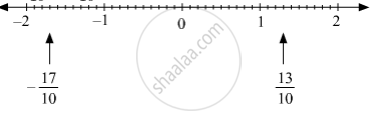SSC (English Medium) Class 8Maharashtra State Board
Share

# Show the Following Numbers on a Number Line. Draw a Separate Number Line for Each Example. 13 10 , − 17 10 - SSC (English Medium) Class 8 - Mathematics

ConceptTo Show Rational Numbers on a Number Line

#### Question

Show the following numbers on a number line. Draw a separate number line for each example.

13/10 , (-17)/10

#### Solution

13/10 , -17/10 can be represented on the number line as follows.Is there an error in this question or solution?

#### APPEARS IN

Balbharati Solution for Balbharati Class 8 Mathematics (2019 to Current)
Chapter 1: Rational and Irrational numbers
Practice Set 1.1 | Q: 1.4 | Page no. 2
Solution Show the Following Numbers on a Number Line. Draw a Separate Number Line for Each Example. 13 10 , − 17 10 Concept: To Show Rational Numbers on a Number Line.
S